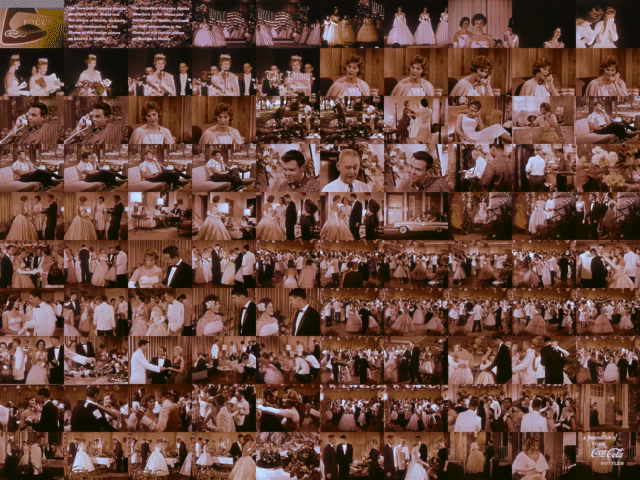# Divide the video into 100 with ffmpeg and display it in 100 divisions on one screen

## Target audience

As shown below, it is intended for those who want to divide the video into 100 and display it on one screen in 100 divisions.## manner

#### `10x10.sh`

``````
## USAGE: sh 10x10.sh input.mp4
ffmpeg -i "\$1" -filter_complex '[0:a]asplit=100'`seq -s '' -f '[a%02g]' 0 99`';[0:v]scale=192:-1,split=100'`seq -s '' -f '[v%02g]' 0 99`';'`ffprobe "\$1" -hide_banner -show_entries format=duration | perl -ne 'next if not m{^duration=(.*)\$}; printf q([v%1\$02d]trim=%2\$f:%3\$f,setpts=PTS-STARTPTS[v%1\$02d];[a%1\$02d]atrim=%2\$f:%3\$f,asetpts=PTS-STARTPTS[a%1\$02d];), \$_, \$1*\$_/100, \$1*(\$_+1)/100 for (00 .. 99);'``seq -s '' -f '[v%02g]' 0 99`'xstack=inputs=100:layout='"`perl -e 'for \$y (0 .. \$ARGV - 1) { \$h = (\$y ? qq(\$h+h0) : 0); for \$x (0 .. \$ARGV - 1) { \$w = (\$x ? qq(\$w+w0) : 0); push @o, qq(\${w}_\$h) } } END { print join q(|), @o }' 10 10`"':shortest=1[v];'`seq -s '' -f '[a%02g]' 0 99`'amix=inputs=100:duration=shortest,loudnorm[a]' -map '[v]' -map '[a]' -shortest output.mp4 -y
``````

If you save the above and run `sh 10x10.sh input.mp4`, you will get ʻoutput.mp4` from ʻinput.mp4`. I’m using version 4.3.1 of `ffmpeg`` ffprobe` and `perl`` seq`. `perl` and` seq` only generate filter statements.

### Process flow

``````ffmpeg
-i 'input.mp4'
-filter_complex '
[0:a]asplit=100[a00](Omission)[a99];
[0:v]scale=192:-1,split=100[v00](Omission)[v99];
[v00]trim=0.000000:18.099510,setpts=PTS-STARTPTS[v00];[a00]atrim=0.000000:18.099510,asetpts=PTS-STARTPTS[a00];
(Omission)
[v99]trim=1791.851490:1809.951000,setpts=PTS-STARTPTS[v99];[a99]atrim=1791.851490:1809.951000,asetpts=PTS-STARTPTS[a99];
[v00](Omission)[v99]xstack=inputs=100:layout=0_0|0+w0_0|(Omission)|0+w0+w0+w0+w0+w0+w0+w0+w0+w0_0+h0+h0+h0+h0+h0+h0+h0+h0+h0:shortest=1[v];
[a00](Omission)[a99]amix=inputs=100:duration=shortest,loudnorm[a]
'
-map '[v]'
-map '[a]'
-shortest
output.mp4
-y
``````

### Processing of video `[v]`

-scale: The output is assumed to be 1920px horizontally, so I set it to 192px here.
-split: Now there are 100.
–Processing for each input: The duration obtained from `ffprobe`, making each video input 1/100 of the original length.

• trim
• setpts
-xstack: Arrange 100 pieces in order from the upper left. Since all inputs are the same size, only w0 and h0 are used for position calculation.

### Processing of audio `[a]`

-asplit: Now there are 100.
–Processing for each input: The duration obtained from `ffprobe`, making each audio input 1/100 of the original length.

• atrim
• asetpts
-amix: Mix 100 audios.
-loudnorm: If you leave it as it is, the sound will be much smaller, so normalize it.